# 10. The Financial Ratio Analysis (Part 2)

## 10.1 – The Leverage Ratios

We touched upon the topic of financial leverage while discussing Return on Equity and the DuPont analysis. The use of leverage (debt) is like a double edged sword.

Well managed companies seek debt if they foresee a situation where, they can deploy the debt funds in an environment which generates a higher return in contrast to the interest payments the company has to makes to service its debt. Do recollect a judicious use of debt to finance assets also increases the return on equity.

However if a company takes on too much debt, then the interest paid to service the debt eats into the profit share of the shareholders. Hence there is a very thin line that separates the good and the bad debt. Leverage ratios mainly deal with the overall extent of the company’s debt, and help us understand the company’s financial leverage better.

We will be looking into the following leverage ratios:

1. Interest Coverage Ratio
2. Debt to Equity Ratio
3. Debt to Asset Ratio
4. Financial Leverage Ratio

So far we have been using Amara Raja Batteries Limited (ARBL) as an example, however to understand leverage ratios, we will look into a company that has a sizable debt on its balance sheet. I have chosen Jain Irrigation Systems Limited (JISL), I would encourage you calculate the ratios for a company of your choice.

Interest Coverage Ratio:
The interest coverage ratio is also referred to as debt service ratio or the debt service coverage ratio. The interest coverage ratio helps us understand how much the company is earning relative to the interest burden of the company. This ratio helps us interpret how easily a company can pay its interest payments. For example, if the company has an interest burden of Rs.100 versus an income of Rs.400, then we clearly know that the company has sufficient funds to service its debt. However a low interest coverage ratio could mean a higher debt burden and a greater possibility of bankruptcy or default.

The formula to calculate the interest coverage ratio:
[Earnings before Interest and Tax / Interest Payment]

The ‘Earnings before Interest and Tax’ (EBIT) is:
EBITDA – Depreciation & Amortization

Let us apply this ratio on Jain Irrigation Limited. Here is the snapshot of Jain Irrigation’s P&L statement for the FY 14, I have highlighted the Finance costs in red: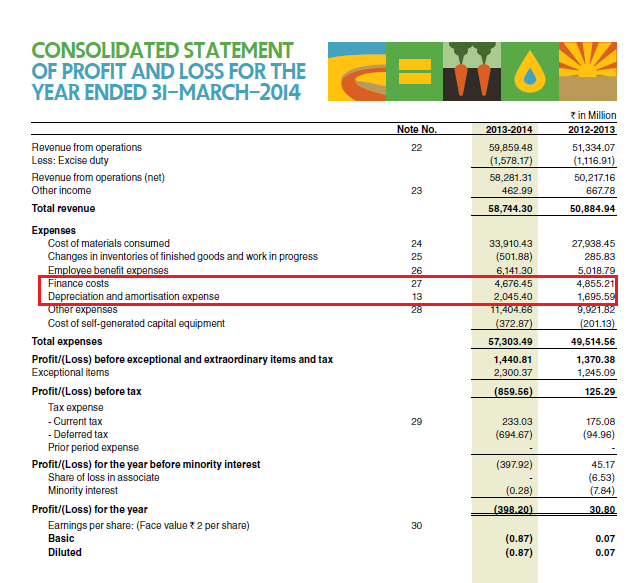We know EBITDA = [Revenue –  Expenses]

To calculate the expenses, we exclude the Finance cost (Rs.467.64Crs) and Depreciation & Amortization cost (Rs.204.54) from the total expenses of Rs.5730.34 Crs.

Therefore EBITDA = Rs.5828.13 – 5058.15 Crs
EBITDA = Rs. 769.98 Crs

We know EBIT = EBITDA – [Depreciation & Amortization]

= Rs.769.98 – 204.54

= Rs. 565.44

We know Finance Cost = Rs.467.64,

Hence Interest coverage is:

= 565.44/ 467.64
= 1.209x

The ‘x’ in the above number represents a multiple. Hence 1.209x should be read as 1.209 ‘times’.

Interest coverage ratio of 1.209x suggests that for every Rupee of interest payment due, Jain Irrigation Limited is generating an EBIT of 1.209 times.

Debt to Equity Ratio:
This is a fairly straightforward ratio. Both the variables required for this computation can be found in the Balance Sheet. It measures the amount of the total debt capital with respect to the total equity capital. A value of 1 on this ratio indicates an equal amount of debt and equity capital. Higher debt to equity (more than 1) indicates higher leverage and hence one needs to be careful. Lower than 1 indicates a relatively bigger equity base with respect to the debt.

The formula to calculate Debt to Equity ratio is:
[Total Debt/Total Equity]

Please note, the total debt here includes both the short term debt and the long term debt.

Here is JSIL’s Balance Sheet, I have highlighted total equity, long term, and short term debt: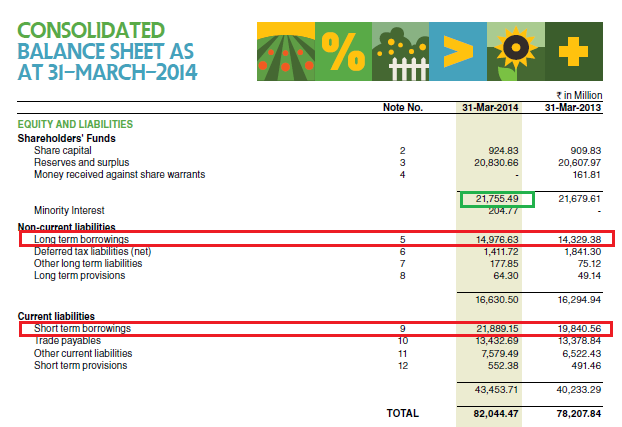Total debt = Long term borrowings + Short term borrowings
= 1497.663 + 2188.915
= Rs.3686.578Crs
Total Equity is Rs.2175.549 Crs

Thus, Debt to Equity ratio will be computed as follows:
= 3686.578 / 2175.549
= 1.69

Debt to Asset Ratio:
This ratio helps us understand the asset financing pattern of the company. It conveys to us how much of the total assets are financed through debt capital.

The formula to calculate the same is:
Total Debt / Total Assets

For JSIL, we know the total debt is Rs.3686.578Crs.
From the Balance Sheet, we know the total assets as Rs.8204.447 Crs: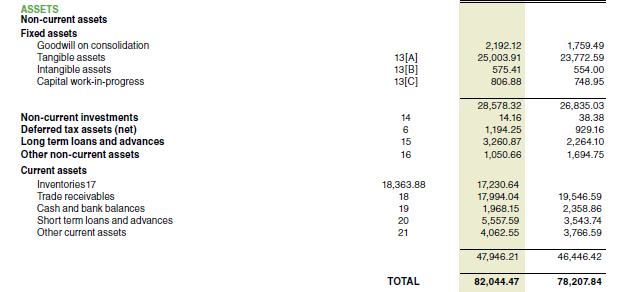Hence the Debt to Asset ratio is:
=3686.578 / 8204.44
= 0.449 or ~45%.

This means roughly about 45% of the assets held by JSIL is financed through debt capital or creditors (and therefore 55% is financed by the owners). Needless to say, higher the percentage the more concerned the investor would be as it indicates higher leverage and risk.

Financial Leverage Ratio
We briefly looked at the financial leverage ratio in the previous chapter, when we discussed about Return on Equity. The financial leverage ratio gives us an indication, to what extent the assets are supported by equity.

The formula to calculate the Financial Leverage Ratio is:
Average Total Asset / Average Total Equity

From JSIL’s FY14 balance sheet, I know the average total assets is Rs.8012.615.The average total equity is Rs.2171.755. Hence the financial leverage ratio or simply the leverage ratio is:
8012.615 / 2171.755
= 3.68

This means JSIL supports Rs.3.68 units of assets for every unit of equity. Do remember higher the number, higher is the company’s leverage.

## 10.2 – Operating Ratios

Operating Ratios also called ‘Activity ratios’ or the ‘Management ratios’ indicate the efficiency of the company’s operational activity. To some degree, the operating ratios reveal the management’s efficiency as well. These ratios are called the Asset Management Ratios, as these ratios indicate the efficiency with which the assets of the company are utilized.Some of the popular Operating Ratios are:

1. Fixed Assets Turnover Ratio
2. Working Capital Turnover Ratio
3. Total Assets Turnover Ratio
4. Inventory Turnover Ratio
5. Inventory Number of Days
6. Receivable Turnover Ratio
7. Days Sales Outstanding (DSO)

The above ratios combine data from both the P&L statement and Balance sheet. We will understand these ratios by calculating them for Amara Raja Batteries Limited.

To get a true sense of how good or bad the operating ratios of a company are, one must compare the ratios with the company’s peers /competitors or these ratios should be compared over the years for the same company.

Fixed Assets Turnover
The ratio measures the extent of the revenue generated in comparison to its investment in fixed assets. It tells us how effectively the company uses its plant and equipment. Fixed assets include the property, plant and equipment. Higher the ratio, it means the company is effectively and efficiently managing its fixed assets.

Fixed Assets Turnover = Operating Revenues / Total Average Asset

The assets considered while calculating the fixed assets turnover should be net of accumulated depreciation, which is nothing but the net block of the company. It should also include the capital work in progress. Also, we take the average assets for reasons discussed in the previous chapter.

From ARBL’s FY14 Balance Sheet: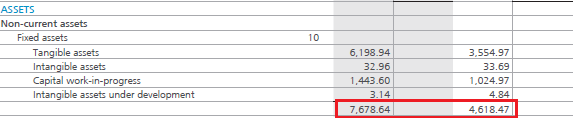= (767.864 + 461.847)/2
= Rs.614.855 Crs
We know the operating revenue for FY14 is Rs.3436.7 Crs, hence the Fixed Asset Turnover ratio is:
= 3436.7 / 614.85
=5.59

While evaluating this ratio, do keep in mind the stage the company is in. For a very well established company, the company may not be utilizing its cash to invest in fixed assets. However for a growing company, the company may invest in fixed assets and hence the fixed assets value may increase year on year. You can notice this in case of ARBL as well, for the FY13 the Fixed assets value is at Rs.461.8 Crs and for the FY14 the fixed asset value is at Rs.767.8 Crs.

This ratio is mostly used by capital intensive industries to analyze how effectively the fixed assets of the company are used.

Working Capital Turnover
Working capital refers to the capital required by the firm to run its day to day operations. To run the day to day operations, the company needs certain type of assets. Typically such assets are – inventories, receivables, cash etc. If you realize these are current assets. A well managed company finances the current assets by current liabilities. The difference between the current assets and current liabilities gives us the working capital of the company.

Working Capital = Current Assets – Current Liabilities

If the working capital is a positive number, it implies that the company has working capital surplus and can easily manage its day to day operations. However if the working capital is negative, it means the company has a working capital deficit. Usually if the company has a working capital deficit, they seek a working capital loan from their bankers.

The concept of ‘Working Capital Management’ in itself is a huge topic in Corporate Finance. It includes inventory management, cash management, debtor’s management etc. The company’s CFO (Chief Financial Officer) strives to manage the company’s working capital efficiently. Of course, we will not get into this topic as we will digress from our main topic.

The working capital turnover ratio is also referred to as Net sales to working capital. The working capital turnover indicates how much revenue the company generates for every unit of working capital. Suppose the ratio is 4, then it indicates that the company generates Rs.4 in revenue for every Rs.1 of working capital. Needless to say, higher the number, better it is. Also, do remember all ratios should be compared with its peers/competitors in the same industry and with the company’s past and planned ratio to get a deeper insight of its performance.

The formula to calculate the Working Capital Turnover:
Working Capital Turnover = [Revenue / Average Working Capital]

Let us implement the same for Amara Raja Batteries Limited. To begin with, we need to calculate the working capital for the FY13 and the FY14 and then find out the average. Here is the snapshot of ARBL’s Balance sheet, I have highlighted the current assets (red) and current liabilities (green) for both the years: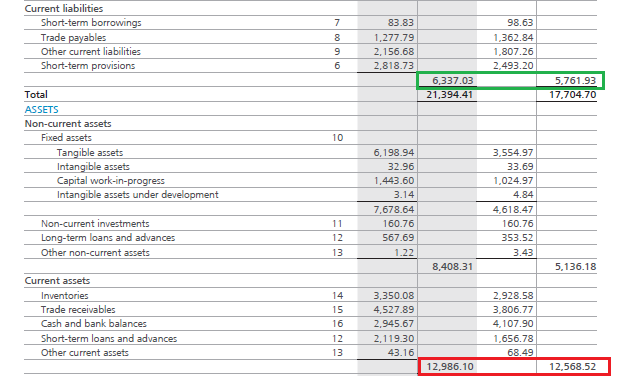The average working capital for the two financial years can be calculated as follows:

 Current Assets for the FY13 Rs.1256.85 Current Liabilities for the FY13 Rs.576.19 Working Capital for the FY13 Rs.680.66 Current Asset for the FY14 Rs.1298.61 Current Liability for the FY14 Rs.633.70 Working Capital for the FY14 Rs.664.91 Average Working Capital Rs.672.78

We know the revenue from operations for ARBL is Rs.3437 Crs. Hence the working capital turnover ratio is:
= 3437 / 672.78
= 5.11 times

The number indicates that for every Rs.1 of working capital, the company is generating Rs.5.11 in terms of revenue. Higher the working capital turnover ratio the better it is, as it indicates the company is generating better sales in comparison with the money it uses to fund the sales.

Total Assets Turnover
This is a very straight forward ratio. It indicates the company’s capability to generate revenues with the given amount of assets. Here the assets include both the fixed assets as well as current assets. A higher total asset turnover ratio compared to its historical data and competitor data means the company is using its assets well to generate more sales.

Total Asset Turnover = Operating Revenue / Average Total Assets

The average total assets for ARBL is as follws –

Total Assets for FY 13 –  Rs.1770.5 Crs and Total Assets for FY 14 – 2139.4 Crs. Hence the average assets would be Rs. 1954.95 Crs.

Operating revenue (FY 14) is Rs. 3437 Crs. Hence Total Asset Turnover is:
= 3437 / 1954.95
= 1.75 times

Inventory Turnover Ratio
Inventory refers to the finished goods that a company maintains in its store or showroom with an expectation of selling the finished goods to prospective clients. Typically, the company besides keeping the goods in the store would also keep some additional units of finished goods in its warehouse.

If a company is selling popular products, then the goods in the inventory gets cleared rapidly, and the company has to replenish the inventory time and again. This is called the ‘Inventory turnover’.

For example think about a bakery selling hot bread. If the bakery is popular, the baker probably knows how many pounds of bread he is likely to sell on any given day. For example, he could sell 200 pounds of bread daily. This means he has to maintain an inventory of 200 pounds of bread every day. So, in this case the rate of replenishing the inventory and the inventory turnover is quite high.

This may not be true for every business. For instance, think of a car manufacturer. Obviously selling cars is not as easy as selling bread. If the manufacturer produces 50 cars, he may have to wait for sometime before he sells these cars. Assume, to sell 50 cars (his inventory capacity) he will need 3 months. This means, every 3 months he turns over his inventory. Hence in a year he turns over his inventory 4 times.

Finally, if the product is really popular the inventory turnover would be high. This is exactly what the ‘Inventory Turnover Ratio’ indicates.

The formula to calculate the ratio is:
Inventory Turnover = [Cost of Goods Sold / Average Inventory]

Cost of goods sold is the cost involved in making the finished good. We can find this in the P&L Statement of the company. Let us implement this for ARBL.

To evaluate the cost of goods sold, I need to look into the expense of the company, here is the extract of the same: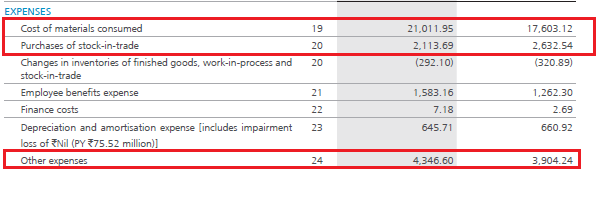Cost of materials consumed is Rs.2101.19 Crs and purchases of stock-in-trade is Rs.211.36 Crs. These line items are directly related to the cost of goods sold. Along with this I would also like to inspect ‘Other Expenses’ to identify any costs that are related to the cost of goods sold. Here is the extract of Note 24, which details ‘Other Expenses’.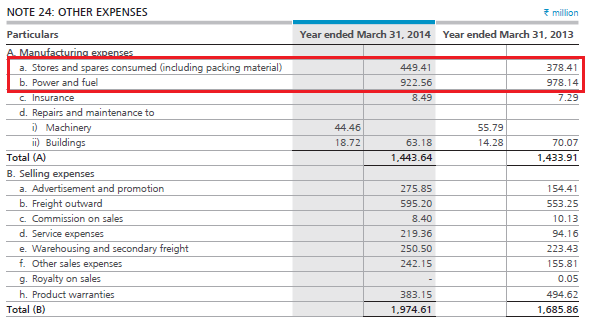There are two expenses that are directly related to manufacturing i.e. Stores & spares consumed which is at  Rs.44.94 Crs and the Power & Fuel cost which is at Rs.92.25Crs.

Hence the Cost of Goods Sold = Cost of materials consumed + Purchase of stock in trade + Stores & spares consumed + Power & Fuel
= 2101.19 + 211.36 + 44.94 + 92.25
COGS= Rs.2449.74 Crs

This takes care of the numerator. For the denominator, we just take the average inventory for the FY13 and FY14. From the balance sheet – Inventory for the FY13 is Rs.292.85 Crs and for the FY14 is Rs.335.00 Crs. The average works out to Rs.313.92 Crs

The Inventory turnover ratio is:
= 2449.74 / 313.92
= 7.8 times
~ 8.0 times a year

This means Amara Raja Batteries Limited turns over its inventory 8 times in a year or once in every 1.5 months. Needless to say, to get a true sense of how good or bad this number is, one should compare it with its competitor’s numbers.

Inventory Number of days
While the Inventory turnover ratio gives a sense of how many times the company  ‘replenishes’ their inventory, the ‘Inventory number of Days’ gives a sense of how much time the company takes to convert its inventory into cash. Lesser the number of days, the better it is. A short inventory number of day’s number implies, the company’s products are fast moving.  The formula to calculate the inventory number of days is:

Inventory Number of Days = 365 / Inventory Turnover

The inventory number of days is usually calculated on a yearly basis. Hence in the formula above, 365 indicates the number of days in a year.

Calculating this for ARBL:
= 365 / 7.8
= 46.79 days
~ 47.0 days

This means ARBL roughly takes about 47 days to convert its inventory into cash. Needless to say, the inventory number of days of a company should be compared with its competitors, to get a sense of how the company’s products are moving.

Now here is something for you to think about – What would you think about the following situation?

1. A certain company under consideration has a high inventory turnover ratio
2. Because of a high inventory turnover ratio, the inventory number of days is very low

On the face of it, the inventory management of this company looks good. A high inventory turnover ratio signifies that the company is replenishing its inventory quickly, which is excellent. Along with the high inventory turnover, a low inventory number of days indicate that the company is quickly able to convert its goods into cash. Again, this is a sign of great inventory management.

However, what if the company has a great product (hence they are able to sell quickly) but a low production capacity? Even in this case the inventory turnover will be high and inventory days will be low.  But a low production capacity can be a bit worrisome as it raises many questions about the company’s production:

1. Why is the company not able to increase their production?
2. Are they not able to increase production because they are short of funds?
3. If they are short of funds, why can’t they seek a bank loan?
4. Have they approached a bank and are not been able to raise a loan successfully?
5. If they are not able to raise a loan, why?
6. What if the management does not have a great track record, hence the banks hesitation to give a loan?
7. If funds are not a problem, why can’t the company increase production?
8. Is sourcing raw materials difficult? Is the raw material required regulated by government (like Coal, power, Oil etc).

As you can see, if any of the points above is true, then a red flag is raised, hence investing in the company may not be advisable. To fully understand the production issues (if any), the fundamental analyst should read through the annual report (especially the management discussion & analysis report) from the beginning to the end.

This means whenever you see impressive inventory numbers, always ensure to double check the production details as well.

Accounts Receivable Turnover Ratio
Having understood the inventory turnover ratio, understanding the receivable turnover ratio should be quite easy. The receivable turnover ratio indicates how many times in a given period the company receives money/cash from its debtors and customers. Naturally a high number indicates that the company collects cash more frequently.

The formula to calculate the same is:
Accounts Receivable Turnover Ratio = Revenue / Average Receivables

From the balance sheet we know,
Trade Receivable for the FY13 : Rs.380.67 Crs
Trade Receivable for the FY14 : Rs. 452.78 Crs
Average Receivable for the FY13 : Rs.416.72
Operating Revenue for the FY14 : Rs.3437 Crs

Hence the Receivable Turnover Ratio is:
= 3437 / 416.72
= 8.24 times a year
~ 8.0 times

This means ARBL receives cash from its customers roughly about 8.24 times a year or once every month and a half.

Days Sales Outstanding (DSO) )/ Average Collection Period/ Day Sales in Receivables
The days sales outstanding ratio illustrates the average cash collection period i.e the time lag between billing and collection. This calculation shows the efficiency of the company’s collection department. Quicker/faster the cash is collected from the creditors, faster the cash can be used for other activities. The formula to calculate the same is:

Days Sales outstanding = 365 / Receivable Turnover Ratio

Solving this for ARBL,
= 365 / 8.24
= 44.29 days

This means ARBL takes about 45 days from the time it raises an invoice to the time it can collect its money against the invoice.

Both Receivables Turnover and the DSO indicate the credit policy of the firm. A efficiently run company, should strike the right balance between the credit policy and the credit it extends to its customers.

### Key takeaways from this chapter

1. Leverage ratios include Interest Coverage, Debt to Equity, Debt to Assets and the Financial Leverage ratios
2. The Leverage ratios mainly study the company’s debt with respect to the company’s ability to service the long term debt
3. Interest coverage ratio inspects the company’s earnings ability (at the EBIT level) as a multiple of its finance costs
4. Debt to equity ratio measures the amount of equity capital with respect to the debt capital. Debt to equity of 1 implies equal amount of debt and equity
5. Debt to Asset ratio helps us understand the asset financing structure of the company (especially with respect to the debt)
6. The Financial Leverage ratio helps us understand the extent to which the assets are financed by the owner’s equity
7. The Operating Ratios also referred to as the Activity ratios include – Fixed Assets Turnover, Working Capital turnover, Total Assets turnover, Inventory turnover, Inventory number of days, Receivable turnover and Day Sales Outstanding ratios
8. The Fixed asset turnover ratio measures the extent of the revenue generated in comparison to its investment in fixed assets
9. Working capital turnover ratio indicates how much revenue the company generates for every unit of working capital
10. Total assets turnover indicates the company’s ability to generate revenues with the given amount of assets
11. Inventory turnover ratio indicates how many times the company replenishes its inventory during the year
12. Inventory number of days represents the number of days the company takes to convert its inventory to cash
1. A high inventory turnover and therefore a low inventory number of days is a great combination
2. However make sure this does not come at the cost of low production capacity
13. The Receivable turnover ratio indicates how many times in a given period the company receives money from its debtors and customers
14. The Days sales outstanding (DSO) ratio indicates the Average cash collection period i.e the time lag between the Billing and Collection

1.Ajit Kumar says:

I was confused on why employee benefits were not counted in COGS. So, I googled COGS and found out this on investopedia

“There are several ways to calculate COGS but one of the more basic ways is to start with the beginning inventory for the period and add the total amount of purchases made during the period, and then deducting the ending inventory. This calculation gives the total amount of inventory or, more specifically, the cost of this inventory, sold by the company during the period. Therefore, if a company starts with \$10 million in inventory, makes \$2 million in purchases and ends the period with \$9 million in inventory, the company’s cost of goods for the period would be \$3 million (\$10 million + \$2 million – \$9 million).”

So for Amaraja Batteries FY13-14, this would look like COGS = (3404+292 -335) = 3359 Crores.

This reduces the GPM significantly. GPM = (3404-3359)/3404 = 1.3%

•Ajit Kumar says:

I am sure I am reading something terribly wrong. Not sure what.

•Ajit Kumar says:

This is the link where I found the alternate way to calculate COGS.
http://www.investopedia.com/terms/c/cogs.asp

•Ajit Kumar says:

I think I found my mistake. Investopedia is suggesting this formula: COGS = Last Year Inventory + Total Expenses – Current Inventory. For ARBL, FY 13-14 this formula is giving COGS = 292 + 2942 – 335 = 2899 Crores. This implies GPM = 3403-E3)/3403 = 14%. Please note that moneycontrol.com also shows a GPM of 14% for ARBL for FY13-14 (http://www.moneycontrol.com/financials/amararajabatteries/ratios/ARB). Your calculation shows a GPM of 28% for ARBL for the same year. I am not sure who is right and who is wrong.

•Karthik Rangappa says:

Ajit – The GMP calculation is shown here – (http://zerodha.com/varsity/chapter/equity-research-part-1/). Calculation of COGS varies a bit from company to company. Also please dont rely on moneycontrol, I’ve found errors multiple times. Suggest you try morningstar India website.

2.Ajit Kumar says:

For a general calculation can’t we use the formula COGS = Current Total Expense + last year inventory – current inventory? I was hoping that this formula works. This would have saved me in looking into each item of expenses and figure out which will go into COGS.

Thanks for your advice regarding moneycontrol. I will check out morningstar now. — Best regards

•Karthik Rangappa says:

Ajit – Maybe I need to explore this a bit deeper.. honestly I’ve never used the formula Investopedia suggests i.e COGS = Current Total Expense + last year inventory – current inventory. In fact more often than not I end up using the one that I have mentioned in the chapter.

•Karthik Rangappa says:

Also on another note, the value that you calculate and the ones mentioned on sites like moneycontrol, morningstar, ET markets etc will hardly ever match point to point…it will differ by few bps. Hence for this reason I always tend to treat these ratios as a ballpark.

•Ajit Kumar says:

The difference were not small. You were speaking of the same ratio GPM in the range of 28% where the investopedia’s formula was giving GPM in the range of 14%, unless I am misinterpreting the formula.

•Karthik Rangappa says:

I understand, like I said I have not used that formula for COGS. Need to dwell more on it. Will surely get back on it as it is learning for me as well.

3.Ajit Kumar says:

There is a typo in the calculation of Fixed Asset Turnover Ratio of ABRL. Since the operating revenue is 3437 and not 353.7, this ratio should be 5.5 and not 0.55.

•Karthik Rangappa says:

Thanks, making the correction now.

4.Ajit Kumar says:

Another typo I guess. Average asset of ABRL for FY2013-14 is 1954 and not 614. Therefore, the total asset turnover should be 1.7 and not 5.5. I hope I am not annoying you.

•Karthik Rangappa says:

Ajit, I rechecked the numbers. Rs.614 Crs seems to be the Average total assets for FY13 and FY14. Unless I’m missing something very obvious !

5.Ajit Kumar says:

Total asset: FY14 = 2139.4, FY13 = 1770.5 Crores. Average = (2139.4 + 1770.5)/2 = 1954.95

•Karthik Rangappa says:

Ah got it 🙂

You are taking the sum total of both Current and Non current assets. However in ‘Fixed Asset’ Turnover ratio, the assets being refereed to is just ‘Fixed Asset” and not all the assets.

•Ajit Kumar says:

No you did not 🙂 The typo I am referring to is in the calculation of Total Asset Turnover ratio. You seem to have calculated Fixed Asset Turnover ratio again.

•Karthik Rangappa says:

My bad ! and I’m quite embarrassed :). Thank you so much for pointing this out, I’ve made the necessary changes.

•Ajit Kumar says:

Thank you. I think I did not emphasize enough in the description about the whereabouts of the error. Anyway …. could you please have these typo related comments deleted now? There purpose is over now.

•Karthik Rangappa says:

I guess the comments should be there, to constantly remind us to be more diligent while proof reading 🙂